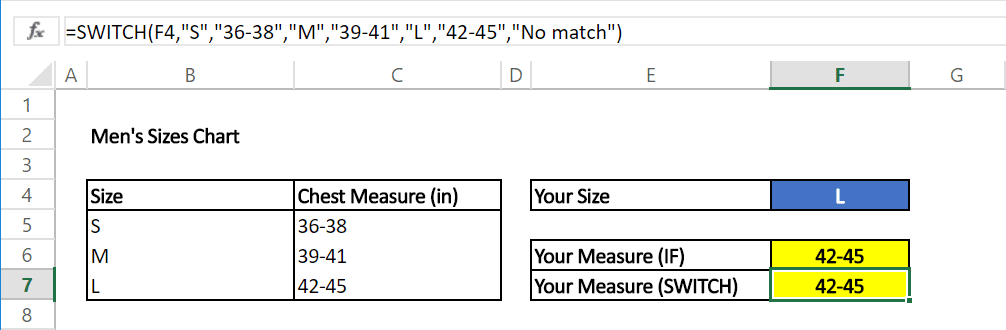Excel case statements can be handled with either SWITCH function or nested IF statements. A popular use for the IF function is creating nested formulas that can check for various criteria. However, nested IF statements can get pretty complicated and cumbersome when dealing with several conditions.

Excel has introduced the SWITCH function which can essentially do what nested IF functions can, using only one function. In this article, we’re going to take a look at the differences between these two Excel case statements and how you can compare several conditions more efficiently. You can download our sample workbook below.

# The Basics of the IF Function

The IF function is one of the most popular functions of Excel. It allows creating conditions, on which a logic can be implemented. The IF function gives a TRUE or FALSE result depending on the outcome of the condition check.

There really are no other alternatives to the IF function, therefore, users typically prefer using nested structures which means using the function over and over again. Let's see how this works on an example. Below is a set of IF formulas inside one another.This formula checks for 4 conditions, "S", "M", "L" and “other” to give a measurement. To do this, we need 2 extra IF functions which are connected to the negative result argument of the previous one. The logic in this structure is to continue the formula if first condition is not met. Then look at the second condition and continue likewise until the criteria is satisfied.As you can imagine, nested IF statements become harder to read and maintain as the number of condition increase. The SWITCH function was introduced as a user-friendly alternative to alleviate the burden of too many IF formulas.

# The Better Alternative: SWITCH Function

The SWITCH function was first introduced in Excel 2016 not to replace the IF function, but instead as an alternative to nested IF formulas. The SWITCH function evaluates the value in its first argument against the value-result pairs, and returns the result from the matched pair if there is a match, or the default value if there are none.

## Syntax

=SWITCH(expression, value1, result1, [default or value2, result2],…[default or value3, result3])

 Argument Description expression Expression is the value (i.e. a number, date, or text) that will be compared against value1…value126. value1…value126 valueN (nth value parameter) is the value that will be compared against an expression. result1…result126 resultN (nth result parameter) is the value to be returned when the corresponding valueN argument that matches the expression. resultN must be entered for each corresponding valueN argument. default (Optional) default is the value to be returned in case there are no matches in any valueN expressions. The default has no corresponding resultN expression. default must be the final argument of the function. If a default value is not defined and no matches are found, the formula returns #N/A error.

Note: Because functions are limited to 254 parameters, you can use up to 126 pairs of value and result arguments.

# SWITCH vs. IF

Let's revisit the measurement example using the SWITCH function this time.The first advantage is the number of formulas used in this argument. When creating a nested IF statement, you need to be actively tracing where you’re at in the formula at each step. Using the SWITCH formula, you can do what 126 IF functions could, using a single function.

Note: It is not advisable to create a SWITCH function that contains 126 value-result pairs. Instead, try using the VLOOKUP function for matching large condition sets. For more information about the VLOOKUP, check out our HOW TO VLOOKUP article.

The second advantage comes from the fact that your formula is going to be much shorter in most cases. Formula text with the SWITCH function is shorter, and easier to read.